Publicité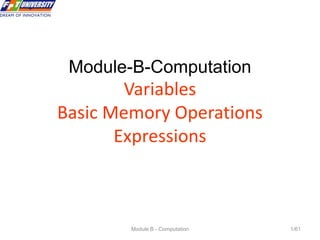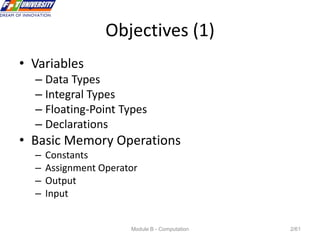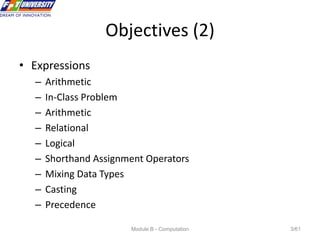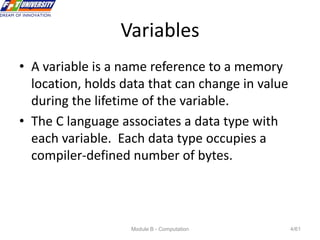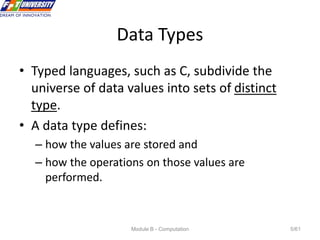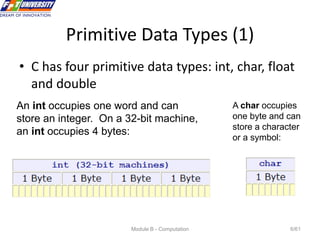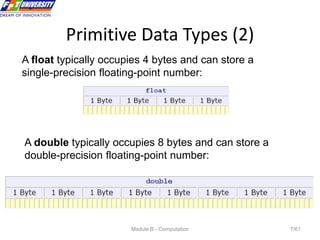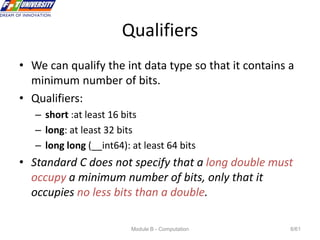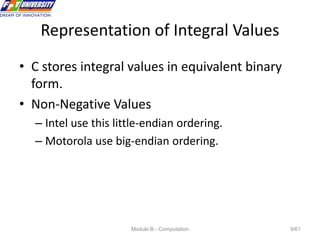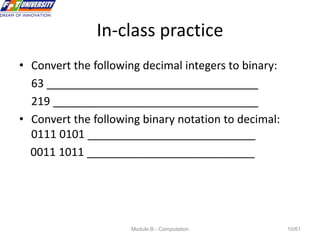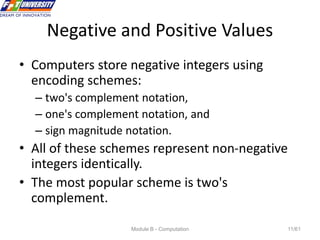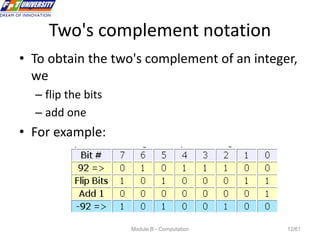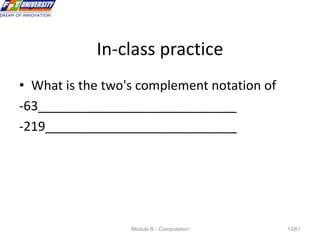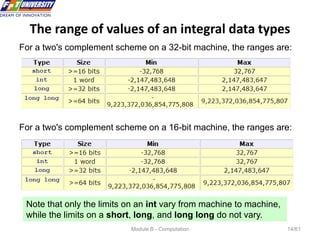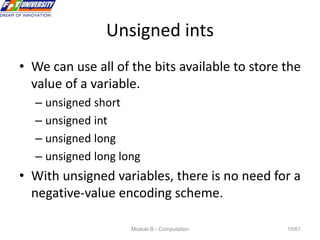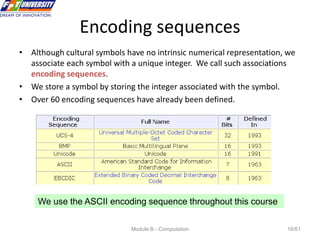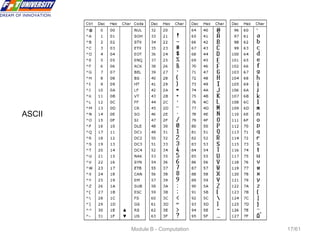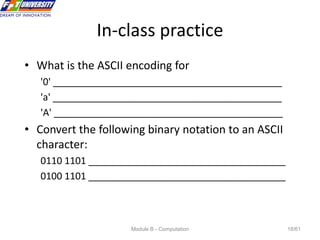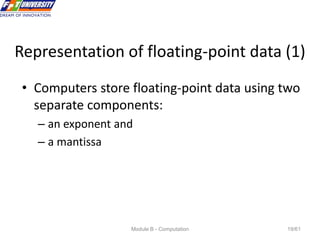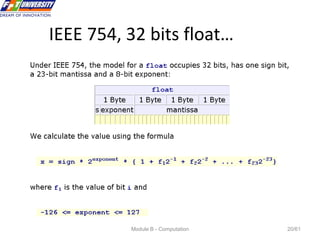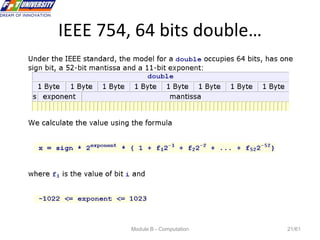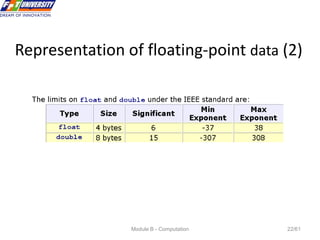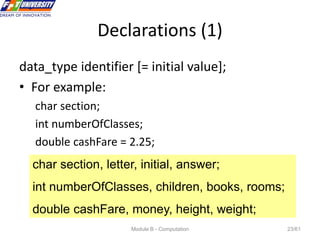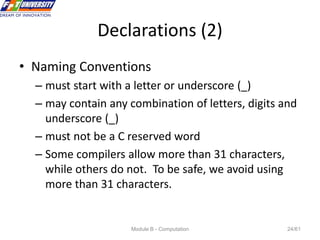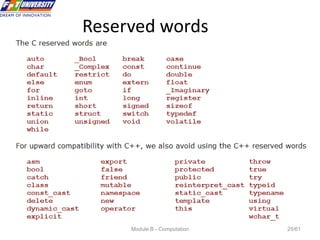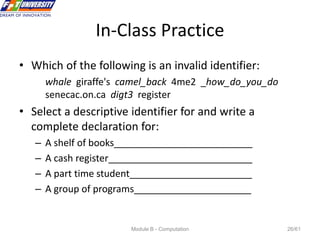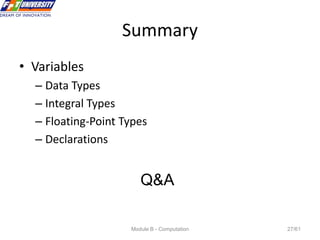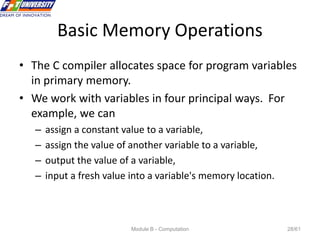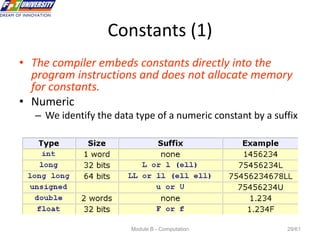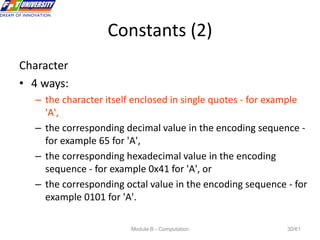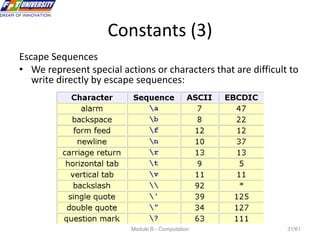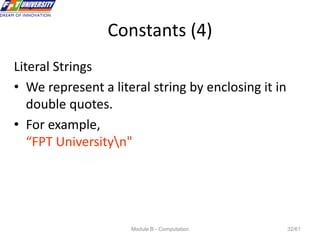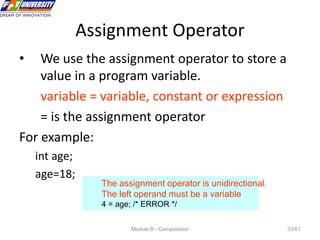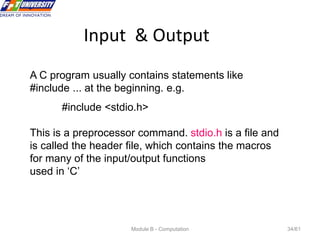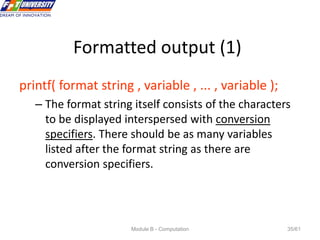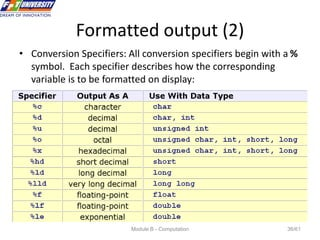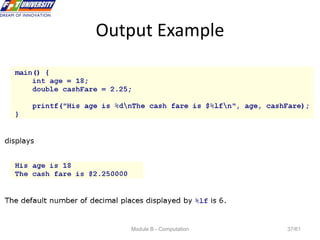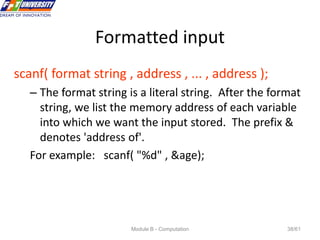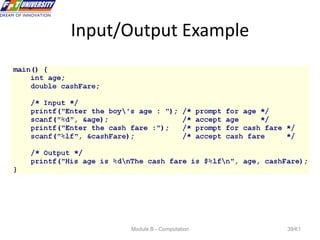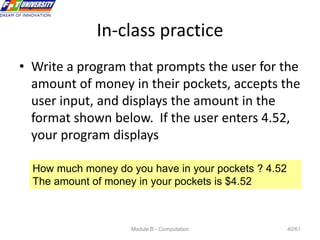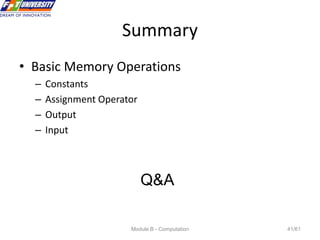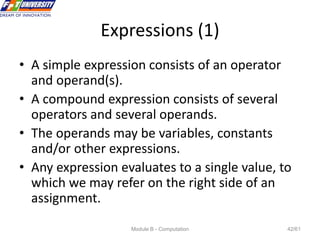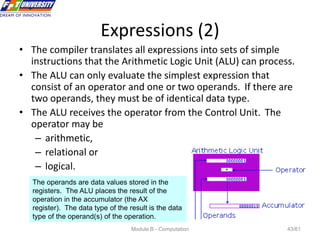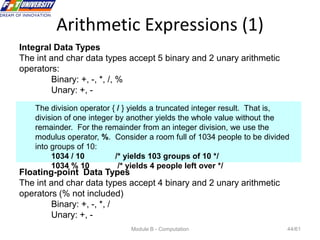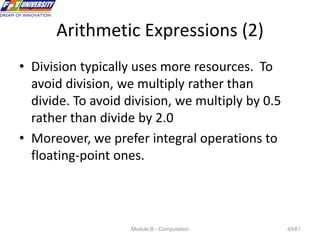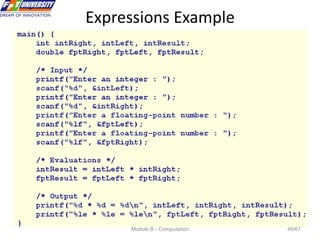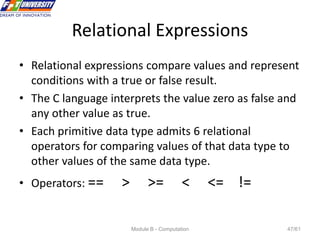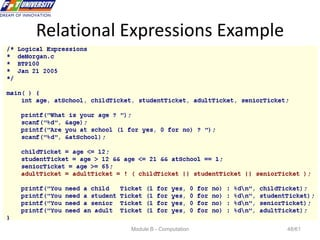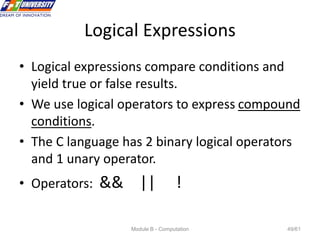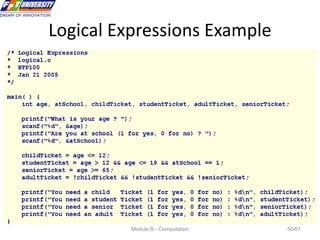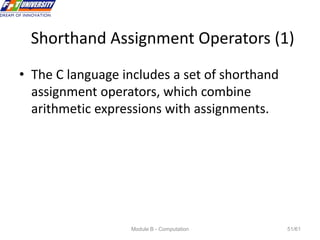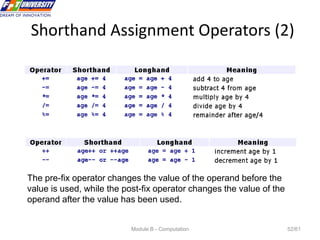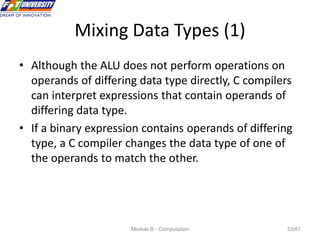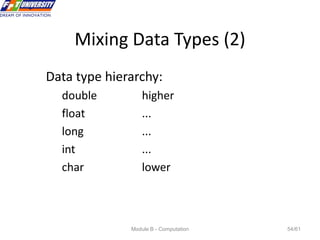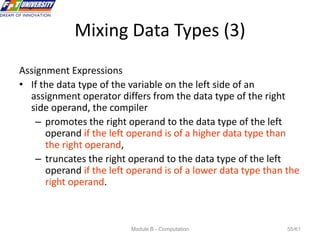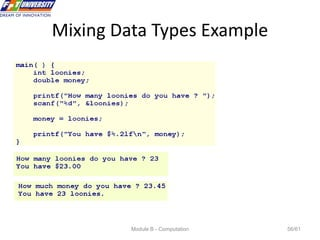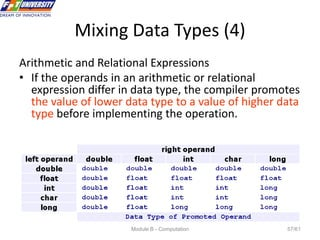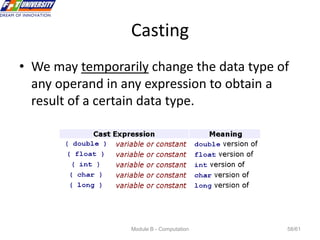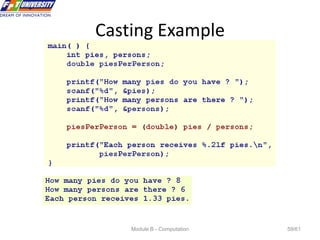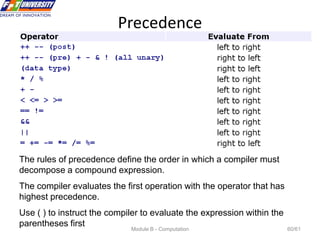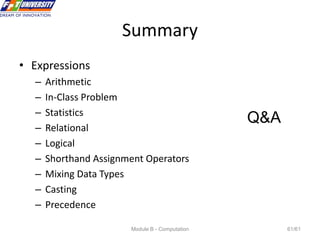1 sur 61
Publicité

### B(02)-Computation.ppt

1. Module B - Computation 1/61 Module-B-Computation Variables Basic Memory Operations Expressions
2. Module B - Computation 2/61 Objectives (1) • Variables – Data Types – Integral Types – Floating-Point Types – Declarations • Basic Memory Operations – Constants – Assignment Operator – Output – Input
3. Module B - Computation 3/61 Objectives (2) • Expressions – Arithmetic – In-Class Problem – Arithmetic – Relational – Logical – Shorthand Assignment Operators – Mixing Data Types – Casting – Precedence
4. Module B - Computation 4/61 Variables • A variable is a name reference to a memory location, holds data that can change in value during the lifetime of the variable. • The C language associates a data type with each variable. Each data type occupies a compiler-defined number of bytes.
5. Module B - Computation 5/61 Data Types • Typed languages, such as C, subdivide the universe of data values into sets of distinct type. • A data type defines: – how the values are stored and – how the operations on those values are performed.
6. Module B - Computation 6/61 Primitive Data Types (1) • C has four primitive data types: int, char, float and double An int occupies one word and can store an integer. On a 32-bit machine, an int occupies 4 bytes: A char occupies one byte and can store a character or a symbol:
7. Module B - Computation 7/61 Primitive Data Types (2) A float typically occupies 4 bytes and can store a single-precision floating-point number: A double typically occupies 8 bytes and can store a double-precision floating-point number:
8. Module B - Computation 8/61 Qualifiers • We can qualify the int data type so that it contains a minimum number of bits. • Qualifiers: – short :at least 16 bits – long: at least 32 bits – long long (__int64): at least 64 bits • Standard C does not specify that a long double must occupy a minimum number of bits, only that it occupies no less bits than a double.
9. Module B - Computation 9/61 Representation of Integral Values • C stores integral values in equivalent binary form. • Non-Negative Values – Intel use this little-endian ordering. – Motorola use big-endian ordering.
10. Module B - Computation 10/61 In-class practice • Convert the following decimal integers to binary: 63 __________________________________ 219 _________________________________ • Convert the following binary notation to decimal: 0111 0101 ___________________________ 0011 1011 ___________________________
11. Module B - Computation 11/61 Negative and Positive Values • Computers store negative integers using encoding schemes: – two's complement notation, – one's complement notation, and – sign magnitude notation. • All of these schemes represent non-negative integers identically. • The most popular scheme is two's complement.
12. Module B - Computation 12/61 Two's complement notation • To obtain the two's complement of an integer, we – flip the bits – add one • For example:
13. Module B - Computation 13/61 In-class practice • What is the two's complement notation of -63____________________________ -219___________________________
14. Module B - Computation 14/61 The range of values of an integral data types For a two's complement scheme on a 32-bit machine, the ranges are: For a two's complement scheme on a 16-bit machine, the ranges are: Note that only the limits on an int vary from machine to machine, while the limits on a short, long, and long long do not vary.
15. Module B - Computation 15/61 Unsigned ints • We can use all of the bits available to store the value of a variable. – unsigned short – unsigned int – unsigned long – unsigned long long • With unsigned variables, there is no need for a negative-value encoding scheme.
16. Module B - Computation 16/61 Encoding sequences • Although cultural symbols have no intrinsic numerical representation, we associate each symbol with a unique integer. We call such associations encoding sequences. • We store a symbol by storing the integer associated with the symbol. • Over 60 encoding sequences have already been defined. We use the ASCII encoding sequence throughout this course
17. Module B - Computation 17/61 ASCII
18. Module B - Computation 18/61 In-class practice • What is the ASCII encoding for '0' ___________________________________________ 'a' ___________________________________________ 'A' ___________________________________________ • Convert the following binary notation to an ASCII character: 0110 1101 _____________________________________ 0100 1101 _____________________________________
19. Module B - Computation 19/61 Representation of floating-point data (1) • Computers store floating-point data using two separate components: – an exponent and – a mantissa
20. Module B - Computation 20/61 IEEE 754, 32 bits float…
21. Module B - Computation 21/61 IEEE 754, 64 bits double…
22. Module B - Computation 22/61 Representation of floating-point data (2)
23. Module B - Computation 23/61 Declarations (1) data_type identifier [= initial value]; • For example: char section; int numberOfClasses; double cashFare = 2.25; char section, letter, initial, answer; int numberOfClasses, children, books, rooms; double cashFare, money, height, weight;
24. Module B - Computation 24/61 Declarations (2) • Naming Conventions – must start with a letter or underscore (_) – may contain any combination of letters, digits and underscore (_) – must not be a C reserved word – Some compilers allow more than 31 characters, while others do not. To be safe, we avoid using more than 31 characters.
25. Module B - Computation 25/61 Reserved words
26. Module B - Computation 26/61 In-Class Practice • Which of the following is an invalid identifier: whale giraffe's camel_back 4me2 _how_do_you_do senecac.on.ca digt3 register • Select a descriptive identifier for and write a complete declaration for: – A shelf of books__________________________ – A cash register___________________________ – A part time student_______________________ – A group of programs______________________
27. Module B - Computation 27/61 Summary • Variables – Data Types – Integral Types – Floating-Point Types – Declarations Q&A
28. Module B - Computation 28/61 Basic Memory Operations • The C compiler allocates space for program variables in primary memory. • We work with variables in four principal ways. For example, we can – assign a constant value to a variable, – assign the value of another variable to a variable, – output the value of a variable, – input a fresh value into a variable's memory location.
29. Module B - Computation 29/61 Constants (1) • The compiler embeds constants directly into the program instructions and does not allocate memory for constants. • Numeric – We identify the data type of a numeric constant by a suffix
30. Module B - Computation 30/61 Constants (2) Character • 4 ways: – the character itself enclosed in single quotes - for example 'A', – the corresponding decimal value in the encoding sequence - for example 65 for 'A', – the corresponding hexadecimal value in the encoding sequence - for example 0x41 for 'A', or – the corresponding octal value in the encoding sequence - for example 0101 for 'A'.
31. Module B - Computation 31/61 Constants (3) Escape Sequences • We represent special actions or characters that are difficult to write directly by escape sequences:
32. Module B - Computation 32/61 Constants (4) Literal Strings • We represent a literal string by enclosing it in double quotes. • For example, “FPT Universityn"
33. Module B - Computation 33/61 Assignment Operator • We use the assignment operator to store a value in a program variable. variable = variable, constant or expression = is the assignment operator For example: int age; age=18; The assignment operator is unidirectional. The left operand must be a variable 4 = age; /* ERROR */
34. Module B - Computation 34/61 Input & Output A C program usually contains statements like #include ... at the beginning. e.g. #include <stdio.h> This is a preprocessor command. stdio.h is a file and is called the header file, which contains the macros for many of the input/output functions used in ‘C’
35. Module B - Computation 35/61 Formatted output (1) printf( format string , variable , ... , variable ); – The format string itself consists of the characters to be displayed interspersed with conversion specifiers. There should be as many variables listed after the format string as there are conversion specifiers.
36. Module B - Computation 36/61 Formatted output (2) • Conversion Specifiers: All conversion specifiers begin with a % symbol. Each specifier describes how the corresponding variable is to be formatted on display:
37. Module B - Computation 37/61 Output Example
38. Module B - Computation 38/61 Formatted input scanf( format string , address , ... , address ); – The format string is a literal string. After the format string, we list the memory address of each variable into which we want the input stored. The prefix & denotes 'address of'. For example: scanf( "%d" , &age);
39. Module B - Computation 39/61 Input/Output Example
40. Module B - Computation 40/61 In-class practice • Write a program that prompts the user for the amount of money in their pockets, accepts the user input, and displays the amount in the format shown below. If the user enters 4.52, your program displays How much money do you have in your pockets ? 4.52 The amount of money in your pockets is \$4.52
41. Module B - Computation 41/61 Summary • Basic Memory Operations – Constants – Assignment Operator – Output – Input Q&A
42. Module B - Computation 42/61 Expressions (1) • A simple expression consists of an operator and operand(s). • A compound expression consists of several operators and several operands. • The operands may be variables, constants and/or other expressions. • Any expression evaluates to a single value, to which we may refer on the right side of an assignment.
43. Module B - Computation 43/61 Expressions (2) • The compiler translates all expressions into sets of simple instructions that the Arithmetic Logic Unit (ALU) can process. • The ALU can only evaluate the simplest expression that consist of an operator and one or two operands. If there are two operands, they must be of identical data type. • The ALU receives the operator from the Control Unit. The operator may be – arithmetic, – relational or – logical. The operands are data values stored in the registers. The ALU places the result of the operation in the accumulator (the AX register). The data type of the result is the data type of the operand(s) of the operation.
44. Module B - Computation 44/61 Arithmetic Expressions (1) The division operator { / } yields a truncated integer result. That is, division of one integer by another yields the whole value without the remainder. For the remainder from an integer division, we use the modulus operator, %. Consider a room full of 1034 people to be divided into groups of 10: 1034 / 10 /* yields 103 groups of 10 */ 1034 % 10 /* yields 4 people left over */ Integral Data Types The int and char data types accept 5 binary and 2 unary arithmetic operators: Binary: +, -, *, /, % Unary: +, - Floating-point Data Types The int and char data types accept 4 binary and 2 unary arithmetic operators (% not included) Binary: +, -, *, / Unary: +, -
45. Module B - Computation 45/61 Arithmetic Expressions (2) • Division typically uses more resources. To avoid division, we multiply rather than divide. To avoid division, we multiply by 0.5 rather than divide by 2.0 • Moreover, we prefer integral operations to floating-point ones.
46. Module B - Computation 46/61 Expressions Example
47. Module B - Computation 47/61 Relational Expressions • Relational expressions compare values and represent conditions with a true or false result. • The C language interprets the value zero as false and any other value as true. • Each primitive data type admits 6 relational operators for comparing values of that data type to other values of the same data type. • Operators: == > >= < <= !=
48. Module B - Computation 48/61 Relational Expressions Example
49. Module B - Computation 49/61 Logical Expressions • Logical expressions compare conditions and yield true or false results. • We use logical operators to express compound conditions. • The C language has 2 binary logical operators and 1 unary operator. • Operators: && || !
50. Module B - Computation 50/61 Logical Expressions Example
51. Module B - Computation 51/61 Shorthand Assignment Operators (1) • The C language includes a set of shorthand assignment operators, which combine arithmetic expressions with assignments.
52. Module B - Computation 52/61 Shorthand Assignment Operators (2) The pre-fix operator changes the value of the operand before the value is used, while the post-fix operator changes the value of the operand after the value has been used.
53. Module B - Computation 53/61 Mixing Data Types (1) • Although the ALU does not perform operations on operands of differing data type directly, C compilers can interpret expressions that contain operands of differing data type. • If a binary expression contains operands of differing type, a C compiler changes the data type of one of the operands to match the other.
54. Module B - Computation 54/61 Mixing Data Types (2) Data type hierarchy: double higher float ... long ... int ... char lower
55. Module B - Computation 55/61 Mixing Data Types (3) Assignment Expressions • If the data type of the variable on the left side of an assignment operator differs from the data type of the right side operand, the compiler – promotes the right operand to the data type of the left operand if the left operand is of a higher data type than the right operand, – truncates the right operand to the data type of the left operand if the left operand is of a lower data type than the right operand.
56. Module B - Computation 56/61 Mixing Data Types Example
57. Module B - Computation 57/61 Mixing Data Types (4) Arithmetic and Relational Expressions • If the operands in an arithmetic or relational expression differ in data type, the compiler promotes the value of lower data type to a value of higher data type before implementing the operation.
58. Module B - Computation 58/61 Casting • We may temporarily change the data type of any operand in any expression to obtain a result of a certain data type.
59. Module B - Computation 59/61 Casting Example
60. Module B - Computation 60/61 Precedence The rules of precedence define the order in which a compiler must decompose a compound expression. The compiler evaluates the first operation with the operator that has highest precedence. Use ( ) to instruct the compiler to evaluate the expression within the parentheses first
61. Module B - Computation 61/61 Summary • Expressions – Arithmetic – In-Class Problem – Statistics – Relational – Logical – Shorthand Assignment Operators – Mixing Data Types – Casting – Precedence Q&A
Publicité